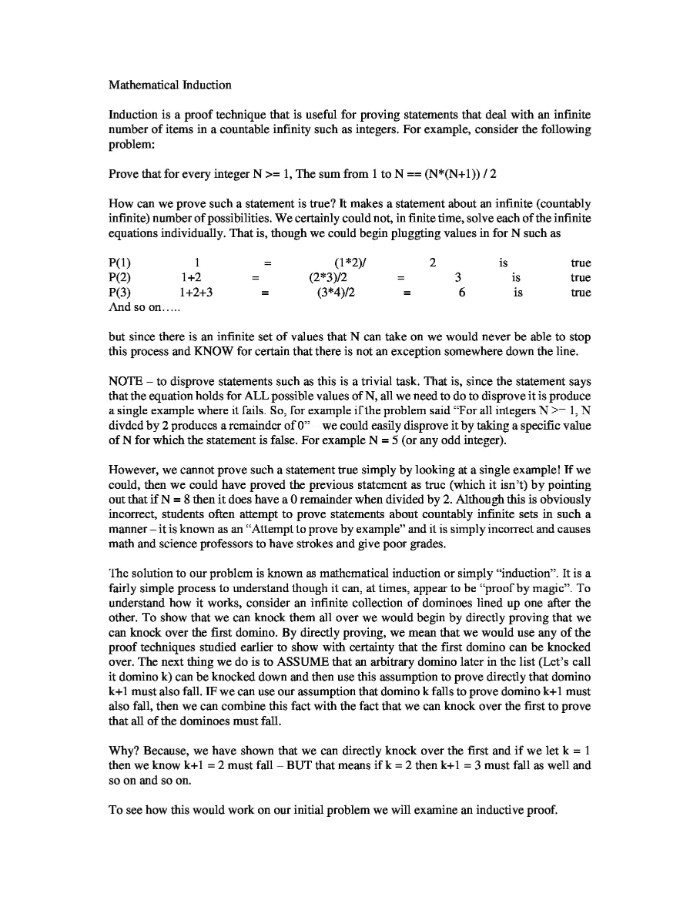# Proving Statements that Deal with an Infinite Number of Items

Home Recent Questions Proving Statements that Deal with an Infinite Number of Items

Mathematical Induction

Induction is a proof technique that is useful for proving statements that deal with an infinite number of items in a countable infinity such as integers. For example, consider the following problem: